# Entire function

(diff) ← Older revision | Latest revision (diff) | Newer revision → (diff)

A function that is analytic in the whole complex plane (except, possibly, at the point at infinity). It can be expanded in a power serieswhich converges in the whole complex plane,.

If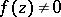everywhere, then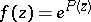, where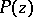is an entire function. If there are finitely many points at whichvanishes and these points are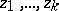(they are called the zeros of the function), then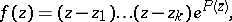whereis an entire function.

In the general case whenhas infinitely many zerosthere is a product representation (see Weierstrass theorem on infinite products)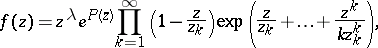(1)

whereis an entire function,if, andis the multiplicity of the zero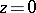if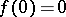.

Let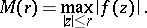If for largethe quantitygrows no faster than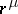, thenis a polynomial of degree not exceeding. Consequently, ifis not a polynomial, thengrows faster than any power of. To estimate the growth ofin this case one takes as a comparison function the exponential function.

By definition,is an entire function of finite order if there is a finite numbersuch that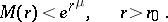The greatest lower boundof the set of numberssatisfying this condition is called the order of the entire function. The order can be computed by the formula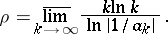Ifof ordersatisfies the condition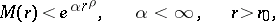(2)

then one says thatis a function of orderand of finite type. The greatest lower boundof the set of numberssatisfying this condition is called the type of the entire function. It is determined by the formula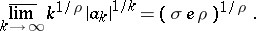Among the entire functions of finite type one distinguishes entire functions of normal type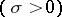and of minimal type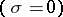. If the condition (2) does not hold for any, then the function is said to be an entire function of maximal type or of infinite type. An entire function of order 1 and of finite type, and also an entire function of order less than 1, characterized by the condition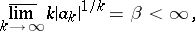is said to be of exponential type.

The zeros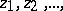of an entire functionof orderhave the property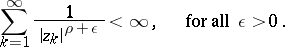Letbe the least integer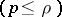such that. Then the following product representation holds (see Hadamard theorem on entire functions)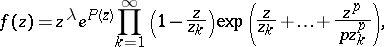(3)

whereis a polynomial of degree not exceeding.

To characterize the growth of an entire functionof finite orderand finite typealong rays, one introduces the quantity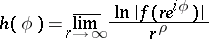— the growth indicator (cf. Growth indicatrix). Here, one always has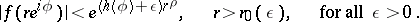If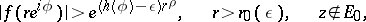where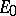is a set which is small in a certain sense (a set of relative measure 0), then the zeros ofare distributed in the plane very regularly in a certain sense, and there is a precise relation betweenand the characteristic (the density) of the zeros. A functionwith this property is said to be a function of completely regular growth.

A function of several variables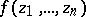is entire if it is analytic for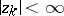(). Again one may introduce the concepts of order and type (conjugate orders and types). A simple representation in the form of an infinite product is not available here, because in contrast to the casethe zeros ofare not isolated.

How to Cite This Entry:
Entire function. Encyclopedia of Mathematics. URL: http://encyclopediaofmath.org/index.php?title=Entire_function&oldid=14495
This article was adapted from an original article by A.F. Leont'ev (originator), which appeared in Encyclopedia of Mathematics - ISBN 1402006098. See original article